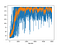# Deriving Policy Gradients and Implementing REINFORCE

Policy gradient methods are ubiquitous in model free reinforcement learning algorithms — they appear frequently in reinforcement learning algorithms, especially so in recent publications. The policy gradient method is also the “actor” part of Actor-Critic methods (check out my post on Actor Critic Methods), so understanding it is foundational to studying reinforcement learning!

Here, we are going to derive the policy gradient step-by-step, and implement the REINFORCE algorithm, also known as Monte Carlo Policy Gradients.

This post assumes some familiarity in reinforcement learning!

# Preliminaries

In essence, policy gradient methods update the probability distribution of actions so that actions with higher expected reward have a higher probability value for an observed state. We will assume discrete (finite) action space and a stochastic (non-deterministic) policy for this post.

1. Reinforcement Learning Objective

The objective function for policy gradients is defined as:

In other words, the objective is to learn a policy that maximizes the cumulative future reward to be received starting from any given time `t` until the terminal time `T`.

Note that `r_{t+1}` is the reward received by performing action `a_{t}` at state `s_{t}` ; `r_{t+1} = R(s_{t}, a_{t})` where `R` is the reward function.

Since this is a maximization problem, we optimize the policy by taking the gradient ascent with the partial derivative of the objective with respect to the policy parameter `theta`.

The policy function is parameterized by a neural network (since we live in the world of deep learning).

2. Expectation

Frequently appearing in literature is the expectation notation — it is used because we want to optimize long term future (predicted) rewards, which has a degree of uncertainty.

The expectation, also known as the expected value or the mean, is computed by the summation of the product of every `x` value and its probability.

Where `P(x)` represents the probability of the occurrence of random variable `x`, and `f(x)`is a function denoting the value of `x`.

Please let me know if there are errors in the derivation!

# Implementing the REINFORCE algorithm

REINFORCE is a Monte-Carlo variant of policy gradients (Monte-Carlo: taking random samples). The agent collects a trajectory τ of one episode using its current policy, and uses it to update the policy parameter. Since one full trajectory must be completed to construct a sample space, REINFORCE is updated in an off-policy way.

Here is the pseudo code for REINFORCE :

So, the flow of the algorithm is:

1. Perform a trajectory roll-out using the current policy
2. Store log probabilities (of policy) and reward values at each step
3. Calculate discounted cumulative future reward at each step
4. Compute policy gradient and update policy parameter
5. Repeat 1–4

We are now going to solve the `CartPole-v0` environment using REINFORCE with normalized rewards*!

Let’s first set up the policy network:

The update function:

*Notice that the discounted reward is normalized (i.e. subtract by mean and divide by the standard deviation of all rewards in the episode). This provides stability in training, and is explained further in Andrej Kaparthy’s post:

“In practice it can can also be important to normalize these. For example, suppose we compute [discounted cumulative reward] for all of the 20,000 actions in the batch of 100 Pong game rollouts above. One good idea is to “standardize” these returns (e.g. subtract mean, divide by standard deviation) before we plug them into backprop. This way we’re always encouraging and discouraging roughly half of the performed actions. Mathematically you can also interpret these tricks as a way of controlling the variance of the policy gradient estimator. A more in-depth exploration can be found here.”

Finally, the main loop:

Results:

Running the main loop, we observe how the policy is learned over 5000 training episodes. Here, we will use the length of the episode as a performance index; longer episodes mean that the agent balanced the inverted pendulum for a longer time, which is what we want to see.

With the y-axis representing the number of steps the agent balances the pole before letting it fall, we see that, over time, the agent learns to balance the pole for a longer duration.Length of episode (Blue) and average length for 10 most recent episodes (orange)

Find the full implementation and write-up on https://github.com/thechrisyoon08/Reinforcement-Learning!

# References:

Andrej Kaparthy’s post: http://karpathy.github.io/2016/05/31/rl/

Official PyTorch implementation in https://github.com/pytorch/examples

Lecture slides from University of Toronto: http://www.cs.toronto.edu/~tingwuwang/REINFORCE.pdf

Written by

Written by